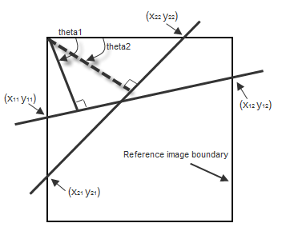# Hough Lines

Find Cartesian coordinates of lines described by rho and theta pairs

## Library

Transforms

`s`## Description

The Hough Lines block finds the points of intersection between the reference image boundary lines and the line specified by a (rho, theta) pair. The block outputs one-based [x y] coordinates for the points of intersection. The boundary lines indicate the left and right vertical boundaries and the top and bottom horizontal boundaries of the reference image.

If the line specified by the (rho, theta) pair does not intersect two border lines in the reference image, the block outputs the values, [`(0,0), (0,0)`]. This output intersection value allows the next block in your model to ignore the points. Generally, the Hough Lines block precedes a block that draws a point or shape at the intersection.

The following figure shows the input and output coordinates for the Hough Lines block.### Port Description

PortInput/OutputSupported Data TypesComplex Values Supported

Theta

Vector of theta values that represent input lines

• Double-precision floating point

• Single-precision floating point

• Fixed point (signed, word length less than or equal to 32)

• 8-, 16-, and 32-bit signed integer

No

Rho

Vector of rho values that represent input lines

Same as Theta port

No

Ref I

Matrix that represents a binary or intensity image or matrix that represents one plane of an RGB image

• Double-precision floating point

• Single-precision floating point

• Fixed-point (signed and unsigned)

• Custom data types

• Boolean

• 8-, 16-, and 32-bit signed integer

• 8-, 16-, and 32-bit unsigned integer

No

Pts

M-by-4 matrix of intersection values, where M is the number of input lines

• 32-bit signed integer

No

## Parameters

Sine value computation method

If you select ```Trigonometric function```, the block computes sine and cosine values to calculate the intersections of the lines during the simulation. If you select `Table lookup`, the block computes and stores the trigonometric values to calculate the intersections of the lines before the simulation starts. In this case, the block requires extra memory.

For floating-point inputs, set the Sine value computation method parameter to ```Trigonometric function```. For fixed-point inputs, set the parameter to ```Table lookup```.

Use this parameter to specify the spacing of the theta-axis. This parameter appears in the dialog box only if, for the Sine value computation method parameter, you select `Table lookup`. parameter appears in the dialog box.

Rounding mode

Select the rounding mode for fixed-point operations.

Overflow mode

Select the overflow mode for fixed-point operations.

Sine table

Choose how to specify the word length of the values of the sine table. The fraction length of the sine table values always equals the word length minus one:

When you select `Specify word length`, you can enter the word length of the sine table.

The sine table values do not obey the Rounding mode and Overflow mode parameters; they saturate and round to `Nearest`.

Product output

Use this parameter to specify how to designate this product output word and fraction lengths:

When you select `Same as first input`, the characteristics match the characteristics of the first input to the block.

When you select `Binary point scaling`, you can enter the word length and the fraction length of the product output, in bits.

When you select `Slope and bias scaling`, you can enter the word length, in bits, and the slope of the product output. All signals in the Computer Vision Toolbox™ blocks have a bias of 0.

See Multiplication Data Types for illustrations depicting the use of the product output.

Accumulator

Use this parameter to specify how you would like to designate the accumulator word and fraction lengths.

When you select `Same as product output` the characteristics match the characteristics of the product output.

When you select `Binary point scaling`, you can enter the Word length and the Fraction length of the accumulator, in bits.

When you select `Slope and bias scaling`, you can enter the Word length, in bits, and the Slope of the Accumulator. All signals in the Computer Vision Toolbox software have a bias of 0.

See Multiplication Data Types for illustrations depicting the use of the accumulator data type in this block.

Lock data type settings against change by the fixed-point tools

Select this parameter to prevent the fixed-point tools from overriding the data types you specify on the block mask. For more information, see `fxptdlg`, a reference page on the Fixed-Point Tool in the Simulink® documentation.

## Examples

The following figure shows Line 1 intersecting the boundaries of the reference image at [```(x11, y11) (x12, y12)```] and Line 2 intersecting the boundaries at [```(x21, y21) (x22, y22)```]Find Local Maxima Computer Vision Toolbox Hough Transform Computer Vision Toolbox